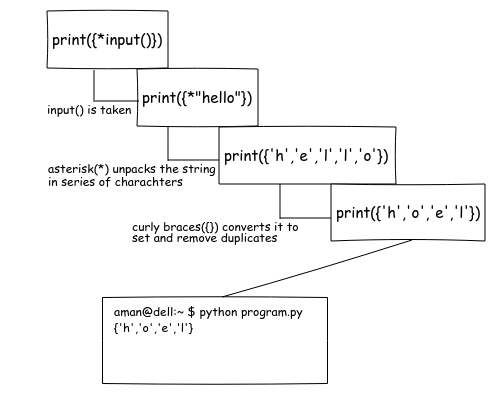# Parsing unique values from input() in Python

Many of the times when we try to solve some programming problems, we need to take input and save only the unique values.

Example:

``````input : aman
output : 'a','m','n'

input : programming
output : 'p','r','o','g','a','m','i','n'
``````

It can also happen on the word level -

``````input : this is this
output : 'this', 'is'
``````

### Solution #1

Iterate over the input and add the item in a pre-initialize list if it is not already there.

``````uv = list()
for i in input():
if not i in uv:
uv.append(i)
print(uv)
``````

### Solution #2

We can do better by using built-in function set(). A set can only contain unique values.

``````inp = set(input())
print(inp)
``````

### Solution #3

It is similar to the previous one. It’s just a shorthand way of writing the previous line.

``````print({*input()})
``````

This is how it works -let’s assume we took “hello” as an input.

We can do the same thing on word level by using the split function.

``````print({*input().split()})

'''
input: hello is hello
output: {'hello','is'}
'''
``````

## Solving CP problem using the above trick

For example, let’s try to solve this problem - Codeforces - Boy or Girl

In short, the problem tells to print “CHAT WITH HER!” If the number of unique characters is even, else print “IGNORE HIM!”.

Constraint - 'a' <= charachter <= 'z'

For example:

``````Input: wjmzbmr
Output: CHAT WITH HER!
``````

Explanation: “wjmzbmr” contains 7 charachters. Here ‘m’ is coming twice, so the unique charachters count is 7-1 = 6. It is an even number so we got the output “CHAT WITH HER!”

``````Input: xiaodao
Output: IGNORE HIM!
``````

Explanation: “xiaodao” contains 7 charachters. ‘a’ and ‘o’ are coming twice, so the unique charachters count is 7-2 = 5. It is an odd number so we got the output “IGNORE HIM!”

#### Solution

• Take input.
• Split it into series of characters.
• Put it into set to remove duplicates.
• Get the length of set.
• If length is even, print “CHAT WITH HER!” else print “IGNORE HIM!”.

#### Code

This is how you can write it in Python -

``````inp = {*input()}
length = len(inp)
if length%2 == 0:
print("CHAT WITH HER!")
else:
print("IGNORE HIM!")
``````

The above code can be written in one line too using idiomatic Python -

``````print("IGNORE HIM!" if len({*input()})%2 else "CHAT WITH HER!")
``````

or

``````print(["CHAT WITH HER!","IGNORE HIM!"][len({*input()})%2])
``````

### Conclusion

“Beautiful is better than ugly.” ~ The Zen of Python, by Tim Peters